# Sequences & Series Questions and Answers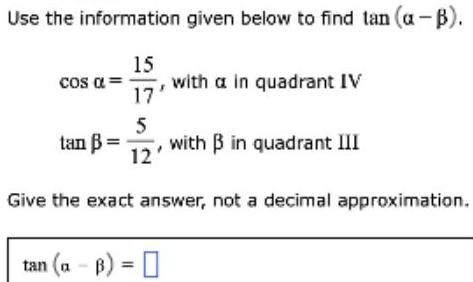Algebra
Sequences & Series
Use the information given below to find tan (a-B). Cos α = 15/17 with a in quadrant IV tan B=5/12 with B in quadrant III Give the exact answer, not a decimal approximation. tan (a-B) =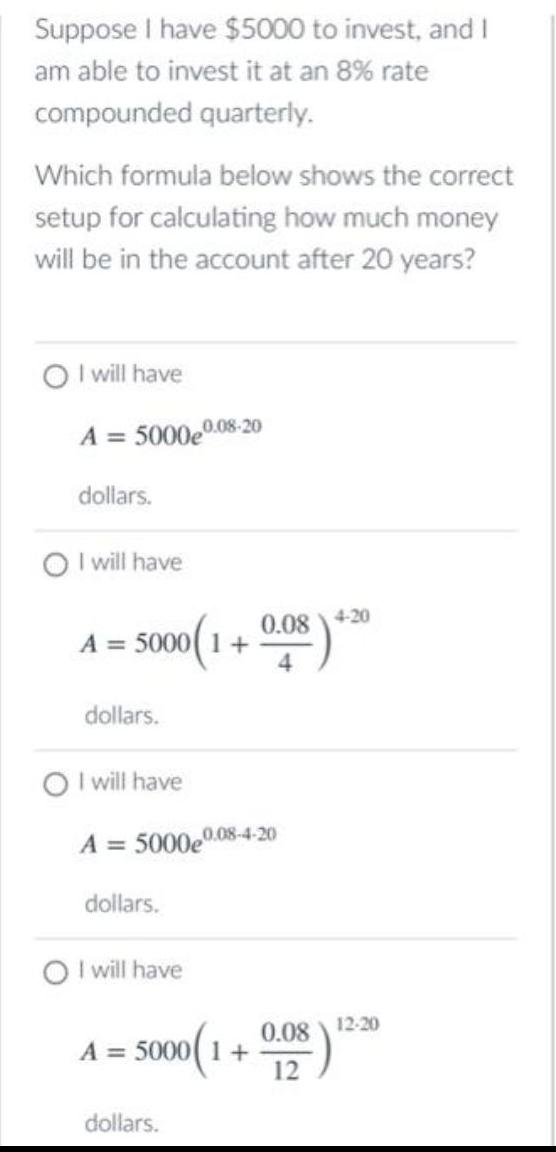Algebra
Sequences & Series
Suppose I have \$5000 to invest, and I am able to invest it at an 8% rate compounded quarterly. Which formula below shows the correct setup for calculating how much money will be in the account after 20 years? I will have A = 5000e0.08-20 dollars. I will have A = 5000(1+0.08/4)4.20 dollars. I will have A = 5000e0.08-4-20 dollars. I will have A = 5000(1+0.08\12)12.20 dollars.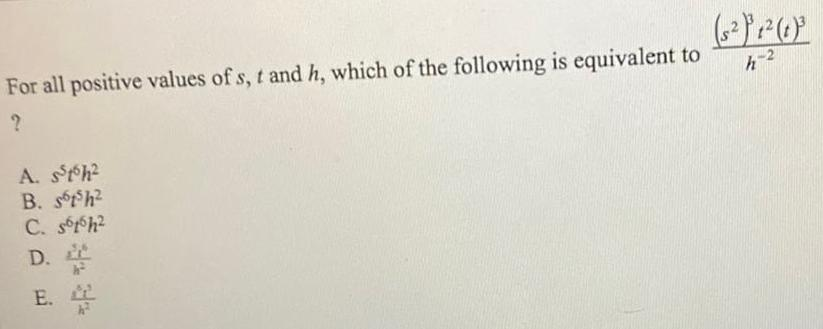Algebra
Sequences & Series
For all positive values of s, t and h, which of the following is equivalent to s23t2t3/h-2? A. s5t6h2 B. s6t5h² C. s6t6h² D.s5t6/h2 E. s6t5/h2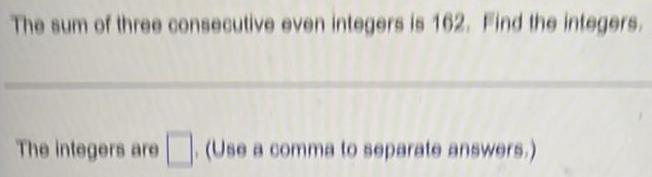Algebra
Sequences & Series
The sum of three consecutive even integers is 162. Find the integers. The integers are (Use a comma to separate answers.)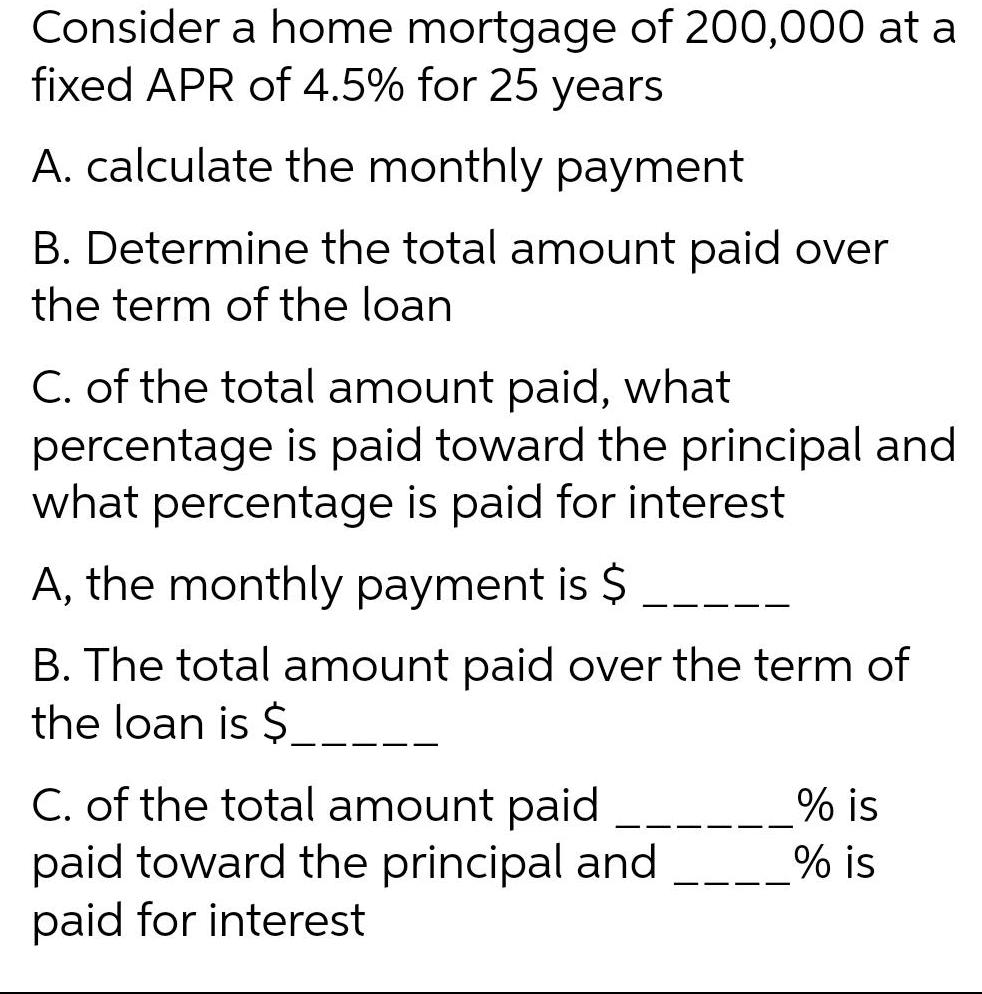Algebra
Sequences & Series
Consider a home mortgage of 200,000 at a fixed APR of 4.5% for 25 years A. calculate the monthly payment B. Determine the total amount paid over the term of the loan C. of the total amount paid, what percentage is paid toward the principal and what percentage is paid for interest A. the monthly payment is \$ B. The total amount paid over the term of the loan is \$ C. of the total amount paid is paid toward the principal and is paid for interest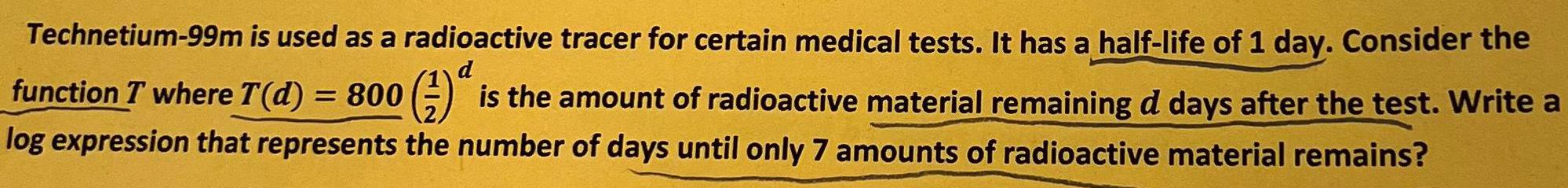Algebra
Sequences & Series
Technetium-99m is used as a radioactive tracer for certain medical tests. It has a half-life of 1 day. Consider the function T where T(d) = 800 (1/2)d is the amount of radioactive material remaining d days after the test. Write a log expression that represents the number of days until only 7 amounts of radioactive material remains?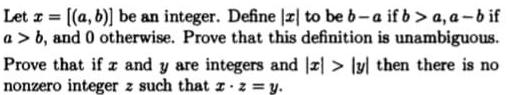Algebra
Sequences & Series
Let z = [(a, b)] be an integer. Define [z] to be b-a if b>a, a-b if a> b, and 0 otherwise. Prove that this definition is unambiguous. Prove that if z and y are integers and z> [y] then there is no nonzero integer z such that x.z = y.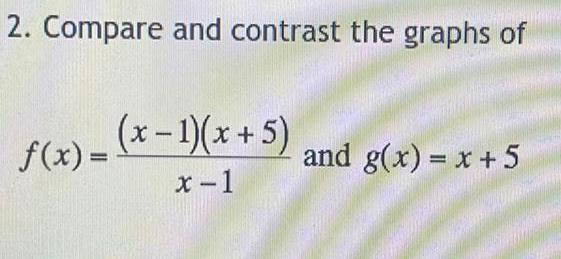Algebra
Sequences & Series
Compare and contrast the graphs of f(x) = (x - 1)(x + 5) / (x-1) and g(x) = x + 5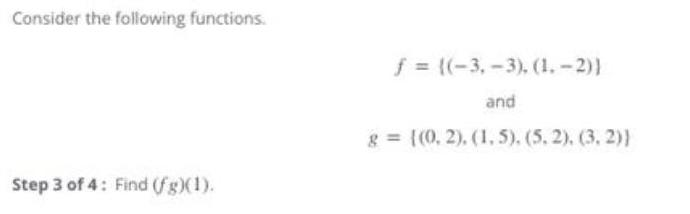Algebra
Sequences & Series
Consider the following functions. f = ((-3,-3), (1.-2)] and g= {(0, 2), (1,5). (5.2), (3, 2)) Step 3 of 4: Find (f g)(1).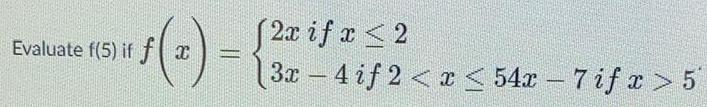Algebra
Sequences & Series
«ƒ(2) = {3+ if " Evaluate f(5) if f x 2x x ≤2 3x-4 if2<x<54x-7 if x > 5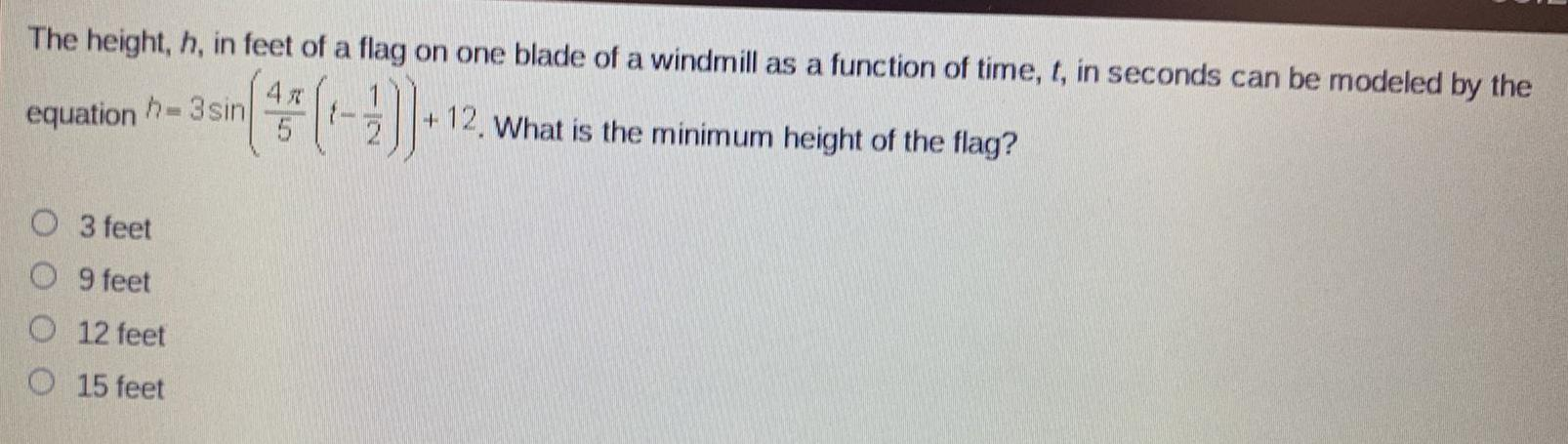Algebra
Sequences & Series
The height, h, in feet of a flag on one blade of a windmill as a function of time, t, in seconds can be modeled by the equation. What is the minimum height of the flag? 3 feet 9 feet 12 feet 15 feet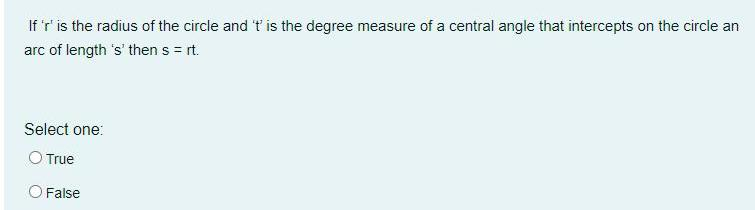Algebra
Sequences & Series
If 'r' is the radius of the circle and 't' is the degree measure of a central angle that intercepts on the circle an arc of length 's' then s = rt. Select one: O True O False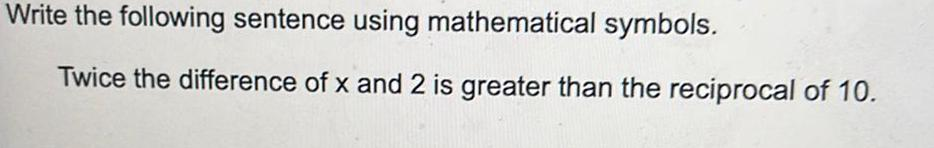Algebra
Sequences & Series
Write the following sentence using mathematical symbols. Twice the difference of x and 2 is greater than the reciprocal of 10.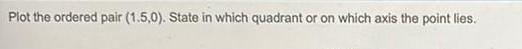Algebra
Sequences & Series
Plot the ordered pair (1.5,0). State in which quadrant or on which axis the point lies.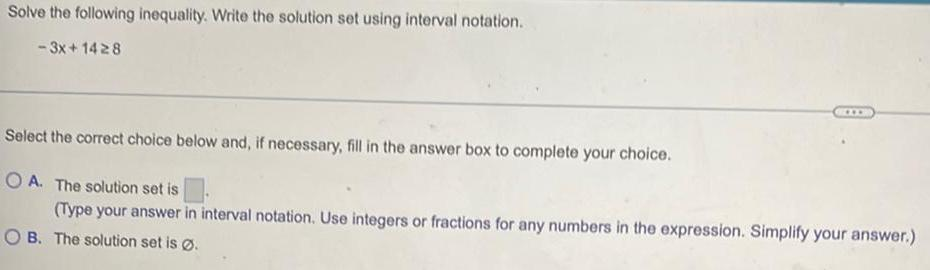Algebra
Sequences & Series
Solve the following inequality. Write the solution set using interval notation. -3x+1428 Select the correct choice below and, if necessary, fill in the answer box to complete your choice. OA. The solution set is (Type your answer in interval notation. Use integers or fractions for any numbers in the expression. Simplify your answer.) OB. The solution set is Ø.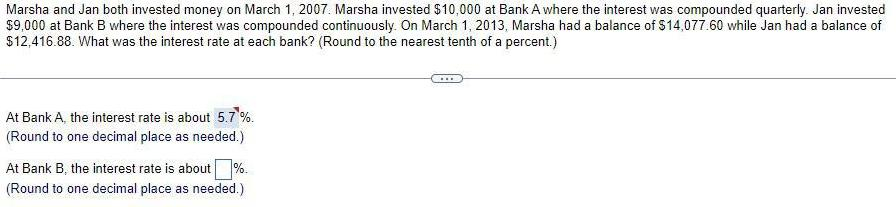Algebra
Sequences & Series
Marsha and Jan both invested money on March 1, 2007. Marsha invested \$10,000 at Bank A where the interest was compounded quarterly. Jan invested \$9,000 at Bank B where the interest was compounded continuously. On March 1, 2013, Marsha had a balance of \$14,077.60 while Jan had a balance of \$12,416.88. What was the interest rate at each bank? (Round to the nearest tenth of a percent.) At Bank A, the interest rate is about 5.7%. (Round to one decimal place as needed.) At Bank B, the interest rate is about %. (Round to one decimal place as needed.) ***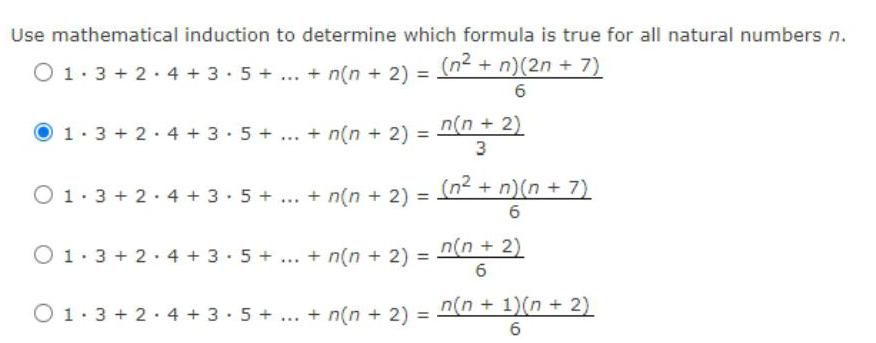Algebra
Sequences & Series
Use mathematical induction to determine which formula is true for all natural numbers n. 01.3 +2.4+3.5 + ... + n(n + 2) = (n² + n)(2n + 7) 6 1 3+2 4+3.5+ O1 3+2 4+3 5+ *** *w* + n(n + 2) = + n(n + 2) = O1 3+2 4+3 5+ ... + n(n+ 2) = n(n + 2) 3 (n² + n)(n+7) 6 n(n + 2) 6 01.3+2 4+3.5+...+ n(n+ 2) = n(n + 1)(n + 2) 6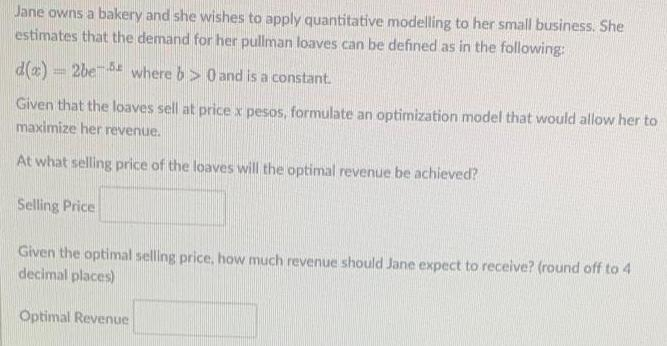Algebra
Sequences & Series
Jane owns a bakery and she wishes to apply quantitative modelling to her small business. She estimates that the demand for her pullman loaves can be defined as in the following: d(x)=2be- where b>0 and is a constant. Given that the loaves sell at price x pesos, formulate an optimization model that would allow her to maximize her revenue. At what selling price of the loaves will the optimal revenue be achieved? Selling Price Given the optimal selling price, how much revenue should Jane expect to receive? (round off to 4 decimal places) Optimal Revenue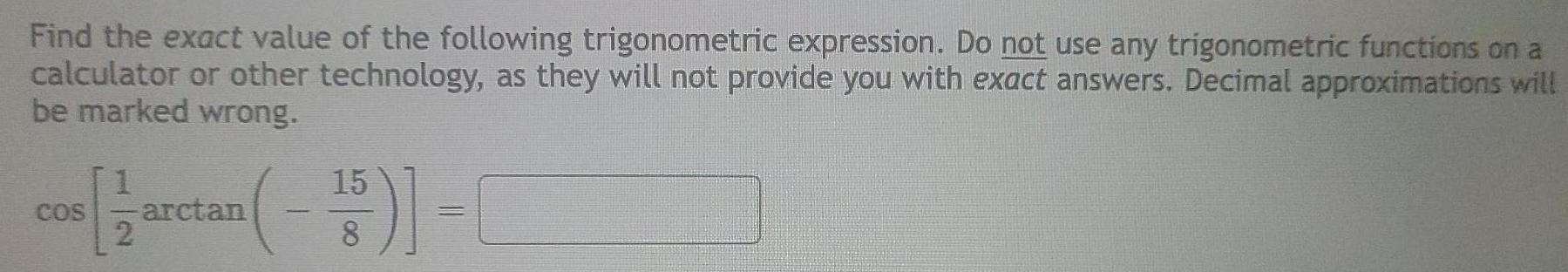Algebra
Sequences & Series
Find the exact value of the following trigonometric expression. Do not use any trigonometric functions on a calculator or other technology, as they will not provide you with exact answers. Decimal approximations will be marked wrong.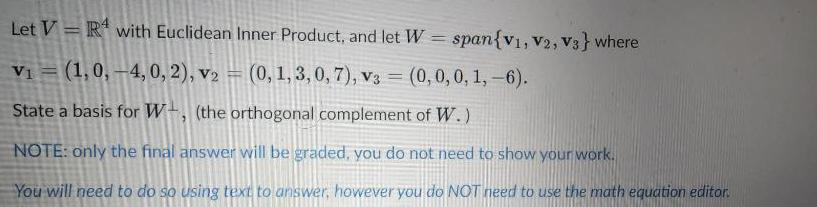Algebra
Sequences & Series
Let VR with Euclidean Inner Product, and let W = span{V1, V2, V3} where V₁ (1,0,-4, 0, 2), v2 = (0, 1, 3, 0, 7), v3 = (0, 0, 0, 1,-6). = State a basis for W, (the orthogonal complement of W.) NOTE: only the final answer will be graded, you do not need to show your work. You will need to do so using text to answer, however you do NOT need to use the math equation editor.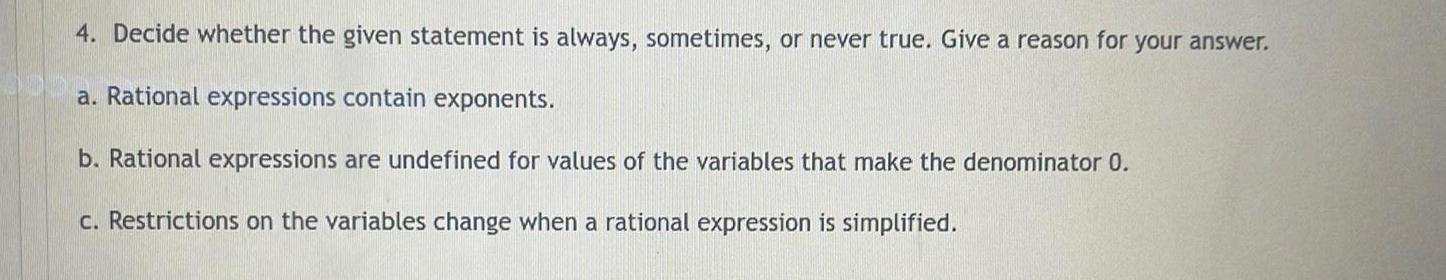Algebra
Sequences & Series
4. Decide whether the given statement is always, sometimes, or never true. Give a reason for your answer. a. Rational expressions contain exponents. b. Rational expressions are undefined for values of the variables that make the denominator 0. c. Restrictions on the variables change when a rational expression is simplified.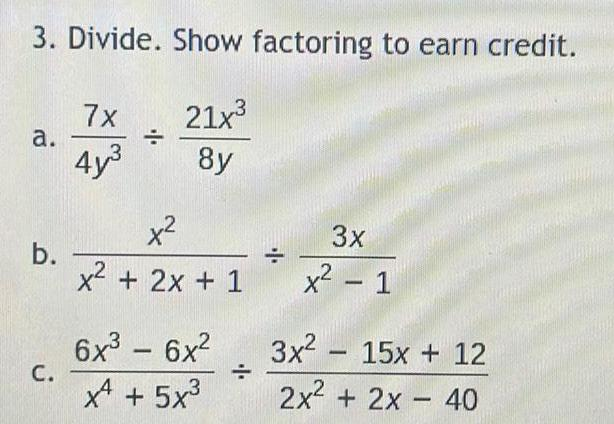Algebra
Sequences & Series
3. Divide. Show factoring to earn credit. 21x³ 8y a. b. C. 7x 4y³ ÷ x² x² + 2x + 1 6x² - 6x² XA +5 x3 ÷ = 3x x² - 1 3x²15x + 12 2x² + 2x - 40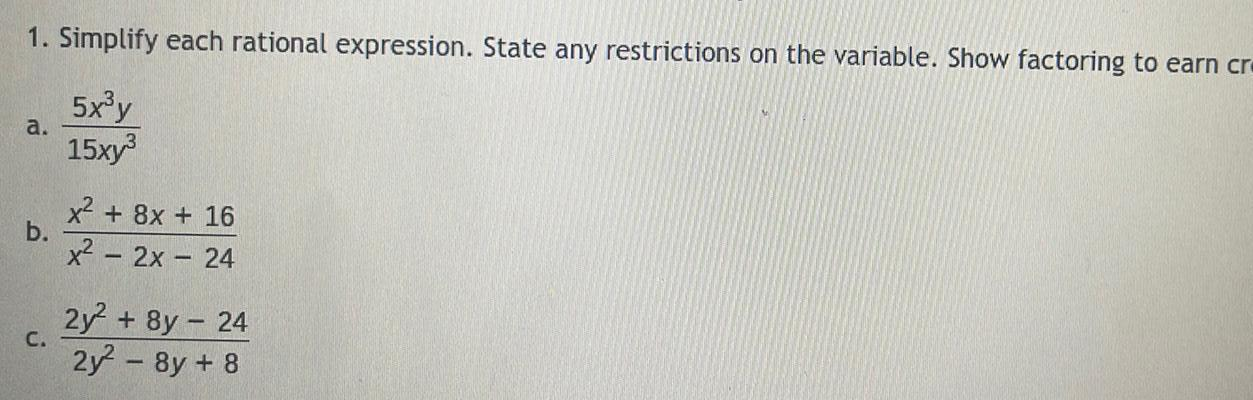Algebra
Sequences & Series
1. Simplify each rational expression. State any restrictions on the variable. Show factoring to earn cr 5x³y 15xy³ a. b. C. x² + 8x + 16 x² - 2x - 24 2y² + 8y-24 2y²2²-8y + 8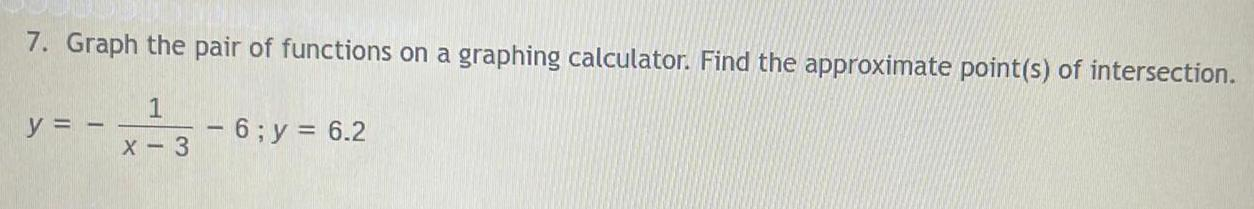Algebra
Sequences & Series
7. Graph the pair of functions on a graphing calculator. Find the approximate point(s) of intersection. y = 1 X-3 - 6;y = 6.2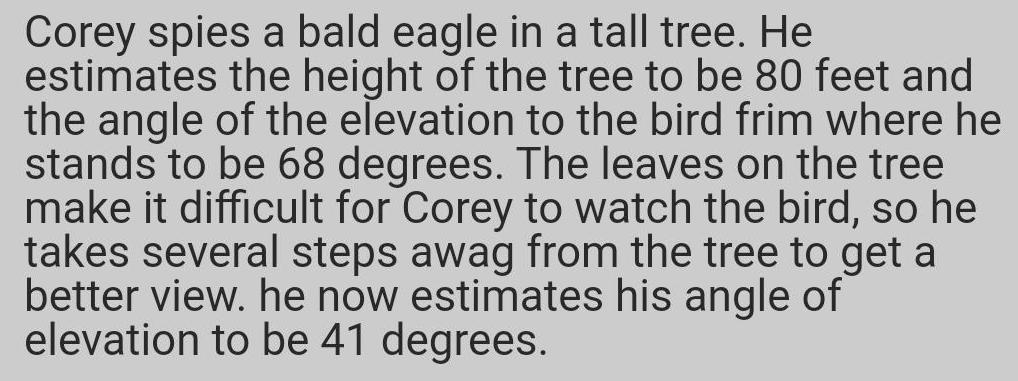Algebra
Sequences & Series
Corey spies a bald eagle in a tall tree. He estimates the height of the tree to be 80 feet and the angle of the elevation to the bird frim where he stands to be 68 degrees. The leaves on the tree make it difficult for Corey to watch the bird, so he takes several steps awag from the tree to get a better view. he now estimates his angle of elevation to be 41 degrees.Algebra
Sequences & Series
2 4. Write an equation for the translation of y = with asymptotes x = 4 and y = -8. X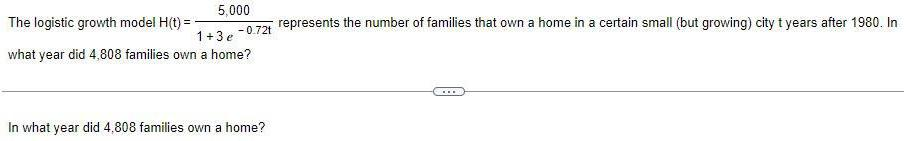Algebra
Sequences & Series
The logistic growth model H(t) = 5,000 1+3e -0.72t what year did 4,808 families own a home? In what year did 4,808 families own a home? represents the number of families that own a home in a certain small (but growing) city t years after 1980. In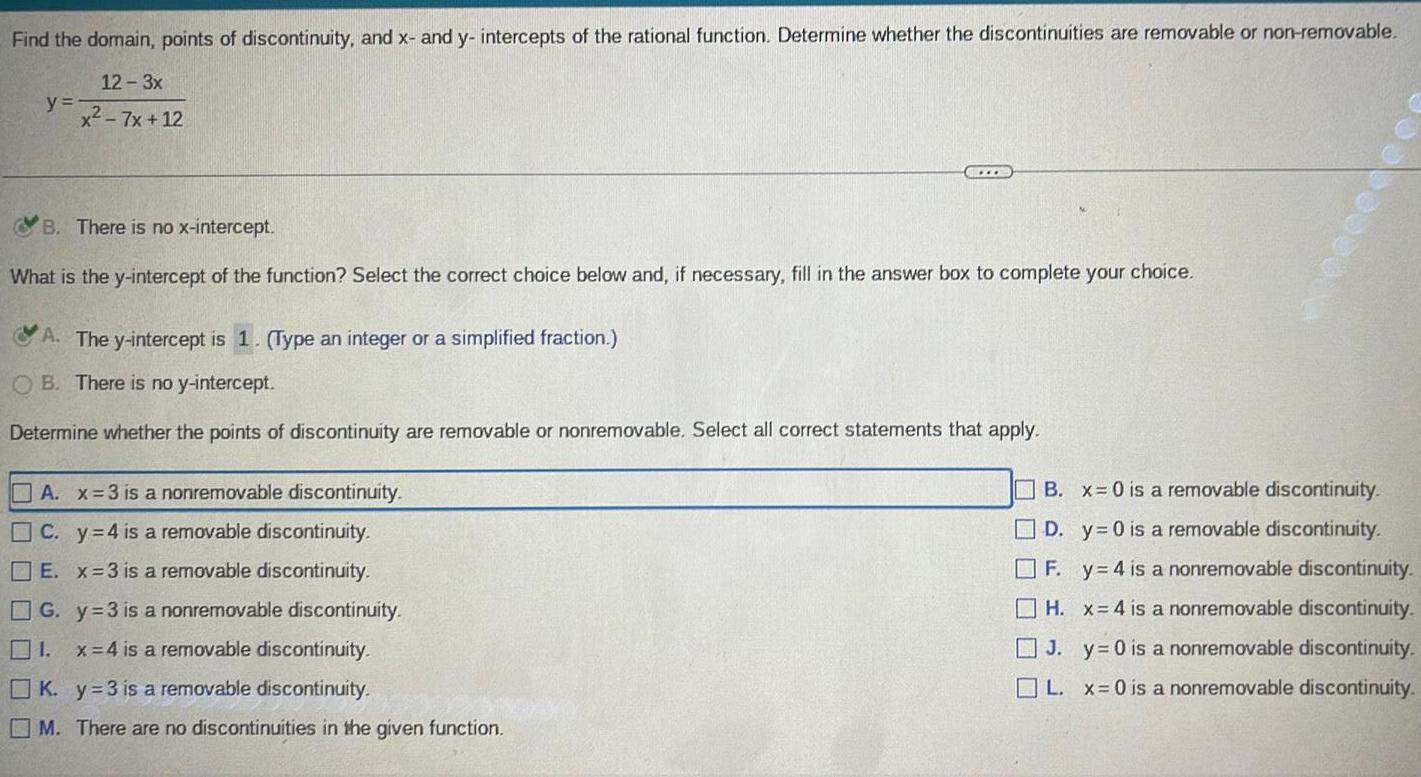Algebra
Sequences & Series
Find the domain, points of discontinuity, and x- and y- intercepts of the rational function. Determine whether the discontinuities are removable or non-removable. y = (12-3x) / (x² - 7x+12) B. There is no x-intercept. What is the y-intercept of the function? Select the correct choice below and, if necessary, fill in the answer box to complete your choice. A. The y-intercept is 1. (Type an integer or a simplified fraction.) OB. There is no y-intercept. Determine whether the points of discontinuity are removable or nonremovable. Select all correct statements that apply. A. x=3 is a nonremovable discontinuity. C. y=4 is a removable discontinuity. E. x=3 is a removable discontinuity. G. y =3 is a nonremovable discontinuity. 1. x=4 is a removable discontinuity. K. y=3 is a removable discontinuity. M. There are no discontinuities in the given function. B. x = 0 is a removable discontinuity. D. y=0 is a removable discontinuity. ]F. y 4 is a nonremovable discontinuity. H. x=4 is a nonremovable discontinuity. y = 0 is a nonremovable discontinuity. 11 J. L. x = 0 is a nonremovable discontinuity.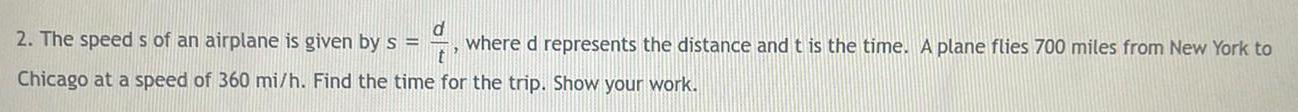Algebra
Sequences & Series
2. The speeds of an airplane is given by s = d where d represents the distance and t is the time. A plane flies 700 miles from New York to t' Chicago at a speed of 360 mi/h. Find the time for the trip. Show your work.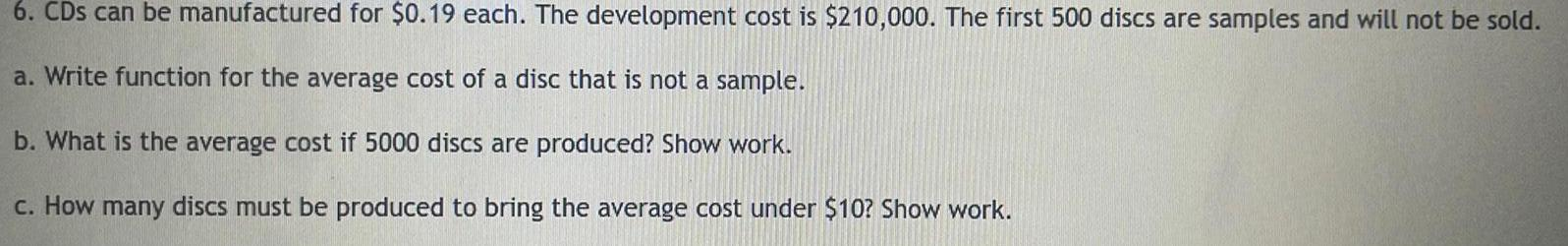Algebra
Sequences & Series
6. CDs can be manufactured for \$0.19 each. The development cost is \$210,000. The first 500 discs are samples and will not be sold. a. Write function for the average cost of a disc that is not a sample. b. What is the average cost if 5000 discs are produced? Show work. c. How many discs must be produced to bring the average cost under \$10? Show work.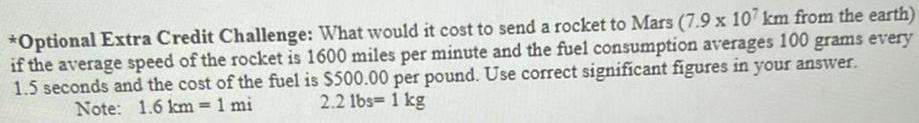Algebra
Sequences & Series
Optional Extra Credit Challenge: What would it cost to send a rocket to Mars (7.9 x 107 km from the earth) if the average speed of the rocket is 1600 miles per minute and the fuel consumption averages 100 grams every 1.5 seconds and the cost of the fuel is \$500.00 per pound. Use correct significant figures in your answer. Note: 1.6 km = 1 mi 2.2 lbs= 1 kg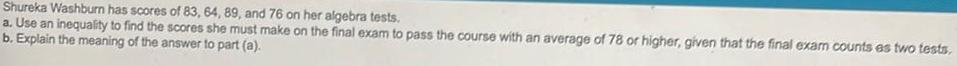Algebra
Sequences & Series
Shureka Washburn has scores of 83, 64, 89, and 76 on her algebra tests. a. Use an inequality to find the scores she must make on the final exam to pass the course with an average of 78 or higher, given that the final exam counts es two tests. b. Explain the meaning of the answer to part (a).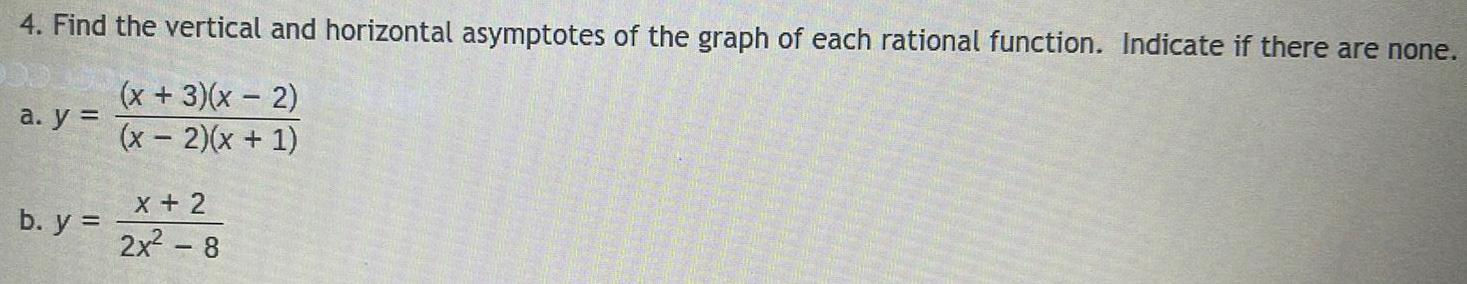Algebra
Sequences & Series
4. Find the vertical and horizontal asymptotes of the graph of each rational function. Indicate if there are none. (x + 3)(x - 2) (x-2)(x + 1) a. y = b. y = x + 2 2x² - 8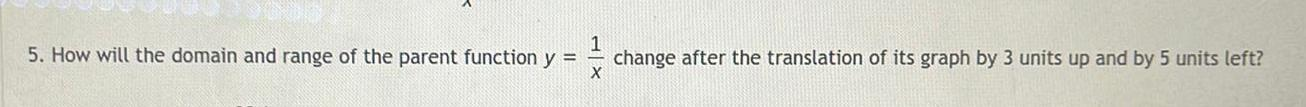Algebra
Sequences & Series
How will the domain and range of the parent function y =1/x change after the translation of its graph by 3 units up and by 5 units left?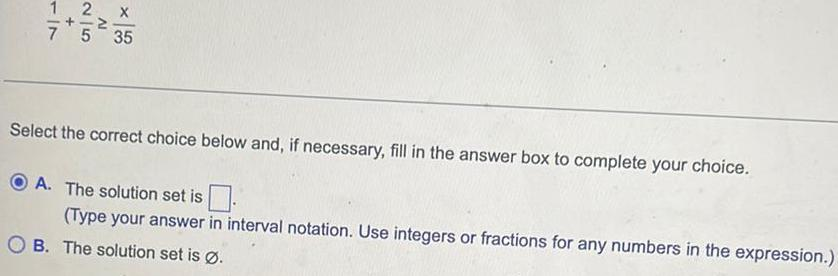Algebra
Sequences & Series
2 AI 7 5 35 Select the correct choice below and, if necessary, fill in the answer box to complete your choice. A. The solution set is (Type your answer in interval notation. Use integers or fractions for any numbers in the expression.) B. The solution set is Ø.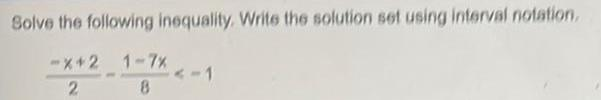Algebra
Sequences & Series
Solve the following inequality. Write the solution set using interval notation. -X+2 1-7% 2 8 <-1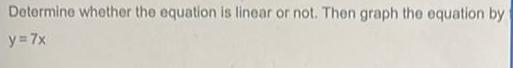Algebra
Sequences & Series
Determine whether the equation is linear or not. Then graph the equation by y = 7x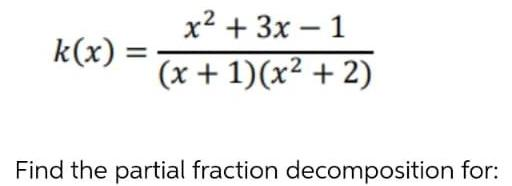Algebra
Sequences & Series
k(x) = x² + 3x - 1 (x + 1)(x² + 2) Find the partial fraction decomposition for: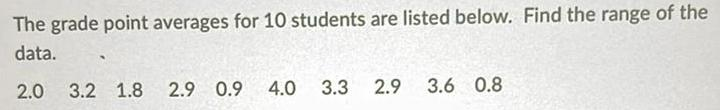Algebra
Sequences & Series
The grade point averages for 10 students are listed below. Find the range of the data. 2.0 3.2 1.8 2.9 0.9 4.0 3.3 2.9 3.6 0.8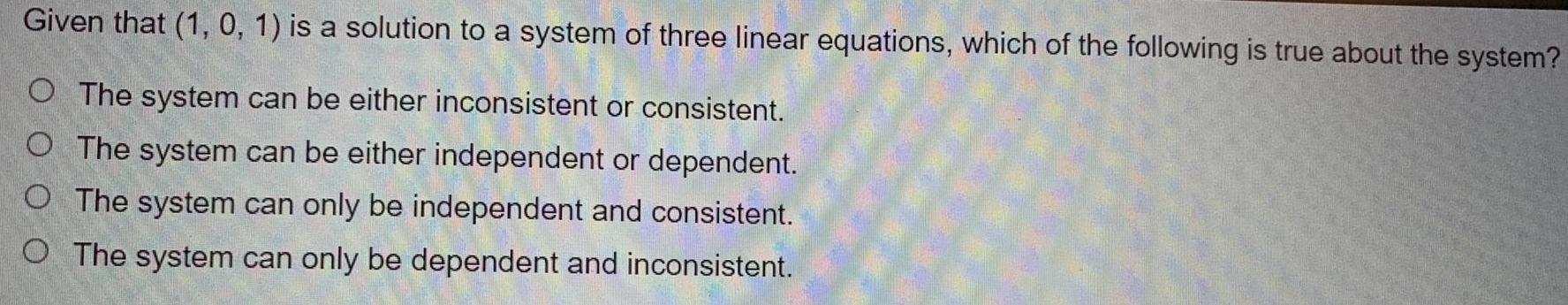Algebra
Sequences & Series
Given that (1, 0, 1) is a solution to a system of three linear equations, which of the following is true about the system? O The system can be either inconsistent or consistent. O The system can be either independent or dependent. O The system can only be independent and consistent. O The system can only be dependent and inconsistent.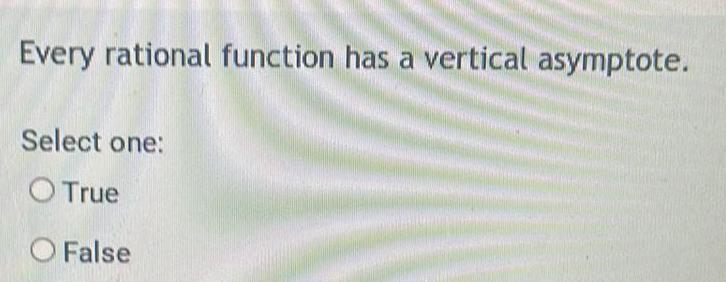Algebra
Sequences & Series
Every rational function has a vertical asymptote. Select one: O True O False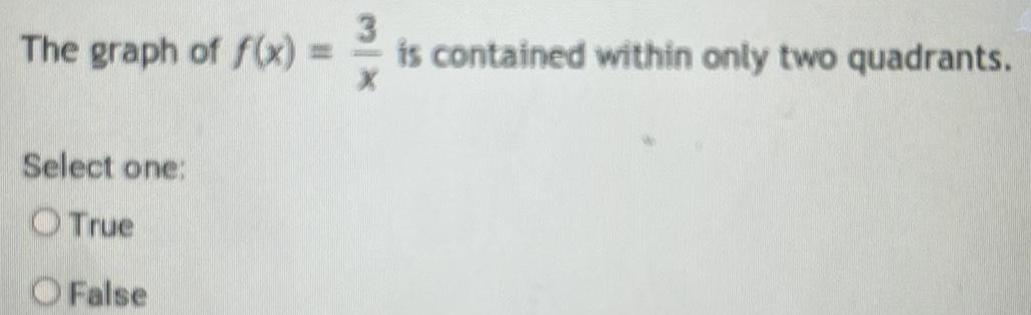Algebra
Sequences & Series
The graph of f(x) = Select one: True O False is contained within only two quadrants.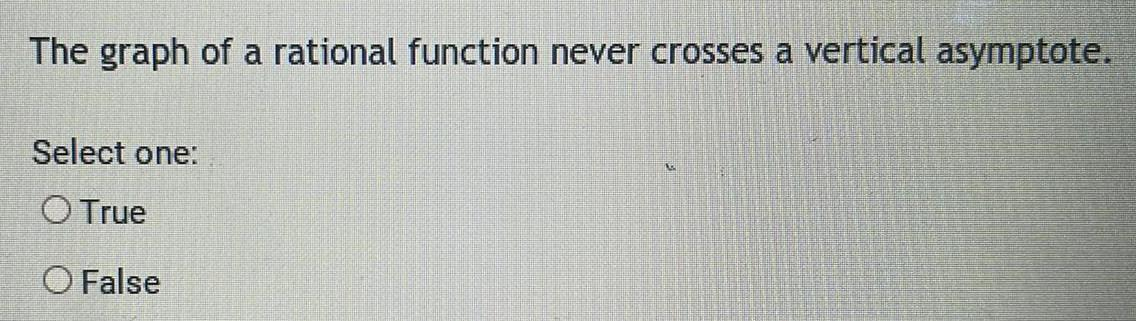Algebra
Sequences & Series
The graph of a rational function never crosses a vertical asymptote. Select one: O True O False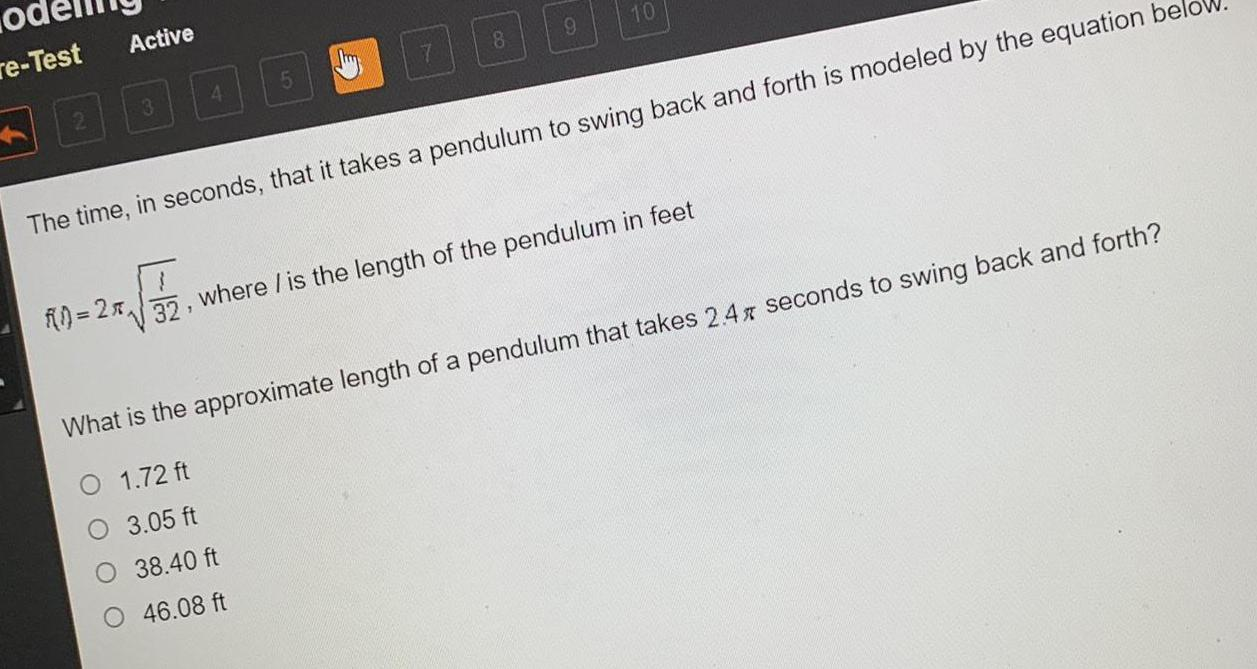Algebra
Sequences & Series
The time, in seconds, that it takes a pendulum to swing back and forth is modeled by the equation be ī 32, where / is the length of the pendulum in feet What is the approximate length of a pendulum that takes 2.4 seconds to swing back and forth? O 1.72 ft 3.05 ft 38.40 ft 46.08 ft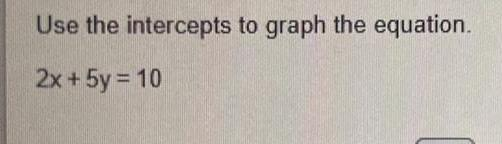Algebra
Sequences & Series
Use the intercepts to graph the equation. 2x + 5y = 10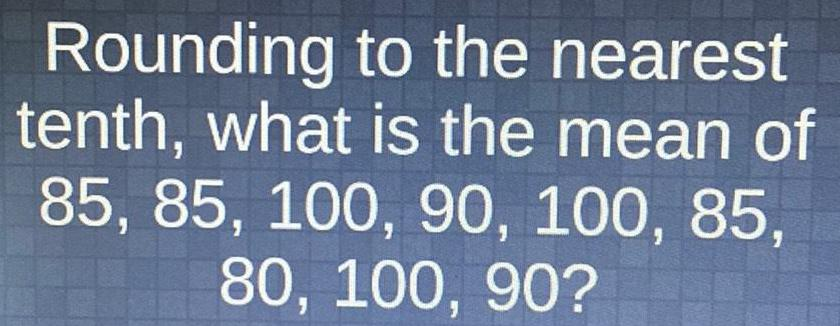Algebra
Sequences & Series
Rounding to the nearest tenth, what is the mean of 85, 85, 100, 90, 100, 85, 80, 100, 90?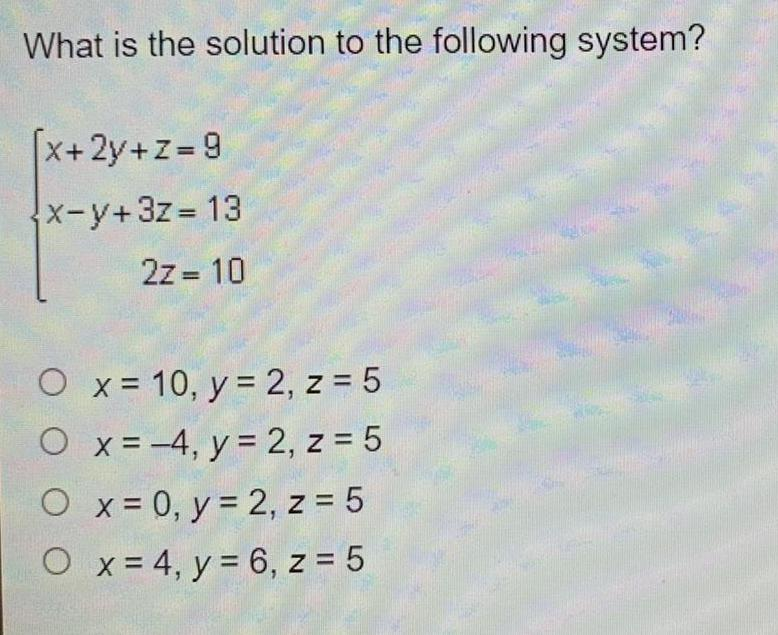Algebra
Sequences & Series
What is the solution to the following system? [x+2y+z= 9 x-y+3z=13 2z = 10 x = 10, y = 2, z = 5 4, y = 2, z = 5 O x = 0, y = 2, z = 5 O x = 4, y = 6, z = 5 O O x = An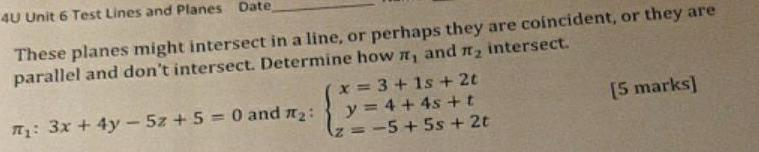Algebra
Sequences & Series
4U Unit 6 Test Lines and Planes Date, These planes might intersect in a line, or perhaps they are coincident, or they are parallel and don't intersect. Determine how ₁ and ₂ intersect. ₁: 3x + 4y - 5z + 5 = 0 and #₂: x=3+1s + 2t y = 4 + 4s+t z = −5+ 5s + 2t [5 marks]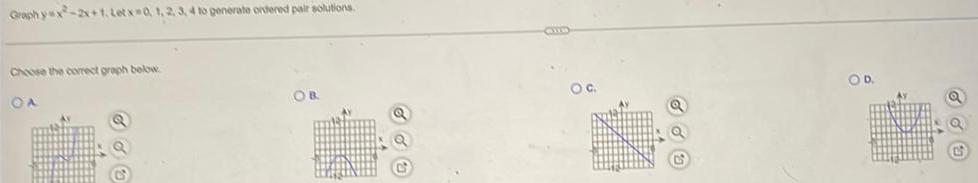Algebra
Sequences & Series
Graphyx-2x+1. Letx=0, 1, 2, 3, 4 to generate ordered pair solutions. Choose the correct graph below A B. C. D.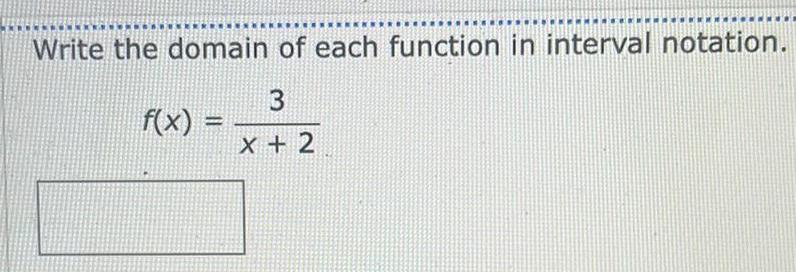Algebra
Sequences & Series
Write the domain of each function in interval notation. 3 X + 2 f(x) =